Prediction of interlayer shear strength parameters for RCC dams using artificial neural network and fuzzy logic system
SHEN Jiarong, XU QianjunState Key Laboratory of Hydroscience and Engineering, Tsinghua University, Beijing 100084, China
Abstract: Predicting the shear strength of roller compacted concrete dams always involves uncertainty and nonlinearities that are influenced by various factors. This study used the artificial neural network and the fuzzy logic system to predict the interlayer shear strength parameters of RCC dams. The data input to the artificial neural network and the fuzzy logic system was divided into data sets from lab tests and data sets from in-situ shear strength tests with five input parameters for the water-binder ratio, total amount of binder, fly ash content, interlayer processing methods and time intervals with two output parameters for f' and c'. The training and testing results show that the lab tests have better correlations than the in-situ tests. Moreover, the artificial neural network has better prediction accuracy than the fuzzy logic system. The predicted values from both the artificial neural network and the fuzzy logic system are in good agreement with the test data. Thus, both models can be used to predict the shear strength parameters and can provide reference predictions for evaluating combinations of layers.
Key words: roller compacted concrete dams     artificial neural networks     fuzzy logic systems     shear strength

1 碾压混凝土层间抗剪断强度

 $\tau=c^{\prime}+\sigma \tan \phi,$ (1)
 $f^{\prime}=\tan \phi.$ (2)

2 人工神经网络 2.1 人工神经网络基本原理

ANN是由大量人工神经元经广泛互连而组成的，它可以用来模拟脑神经系统的结构和功能。人工神经网络具有很强的自适应能力、学习能力、容错能力和鲁棒性，近年来在众多工程领域取得了成功的应用。因此，将人工神经网络方法应用于混凝土抗剪断强度参数的预测，在一定程度上可以克服由于问题的不确定性所导致的困难，具有良好的发展前景。

BP神经网络是一种多层前馈网络，它是至今应用最为普遍的人工神经网络学习算法，据统计有近90%的人工神经网络应用是基于BP算法的。BP神经网络的基本原理是梯度最速下降法，它的中心思想是调整权值使网络总误差最小。BP神经网络由输入层、隐含层和输出层构成。图 1所示为具有输入层、输出层和一个隐含层的BP神经网络的典型结构。图 1中输入节点有M个，输出节点有L个，网络的隐含层有q个神经元。其中：x1, x2, …, xM为网络的实际输入值，y1, y2, …, yL为网络的实际输出值，tk(k=1, 2, …, L)为网络的目标输出值，ek(k=1, 2, …, L)为网络的输出误差。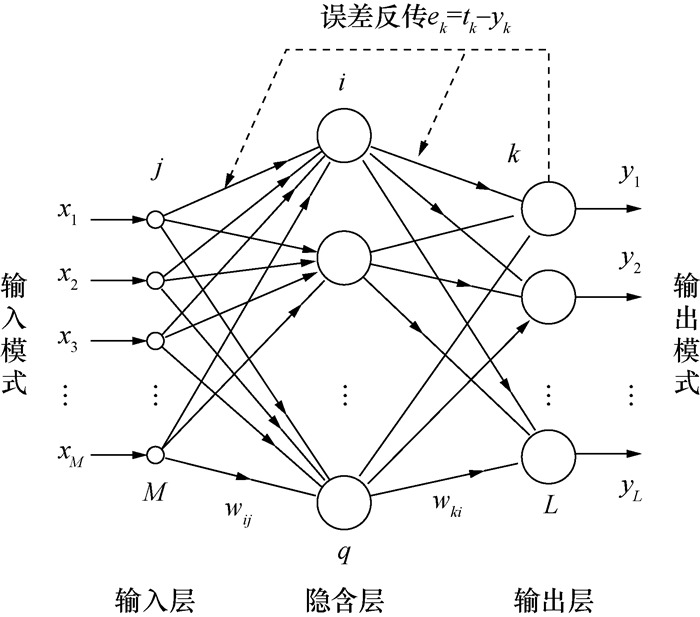图 1 BP网络结构图

2.2 人工神经网络模型

1) 定义及导入训练样本数据。

BP网络需要建立一个训练集进行网络的训练学习，同时还需建立一个测试集来评估已训练好的网络的泛化能力。本文搜集室内试验数据39组，从中随机抽取30组作为训练集，9组作为测试集。原位试验数据52组，从中随机抽取36组作为训练集，16组作为测试集。同时，为了提高人工神经网络的训练速度，对输入样本进行归一化处理，使得输入数值在0~1之间。归一化采用式(3)，

 $y=\frac{x-x_{\min }}{x_{\max }-x_{\min }}.$ (3)

 序号 输入值 期望输出 网络输出 类型 水胶比 胶凝材料含量 粉煤灰掺量 间隔时间 处理方式 f′ c′ f′1 c′1 kg·m-3 % h 1 0.42 190 54 6 0 1.3 3.4 1.4 3.1 训练 2 0.6 141 60 8 0 1.3 2.0 1.3 1.9 样本 … … … … … … … … … … 1 0.45 191 45 20 0 1.1 1.3 1.1 1.4 测试 2 0.55 153 60 6 1 1.2 1.6 1.2 1.6 样本 … … … … … … … … … …

2) 创建人工神经网络。图 2 人工神经网络结构图

3 模糊逻辑系统 3.1 模糊逻辑原理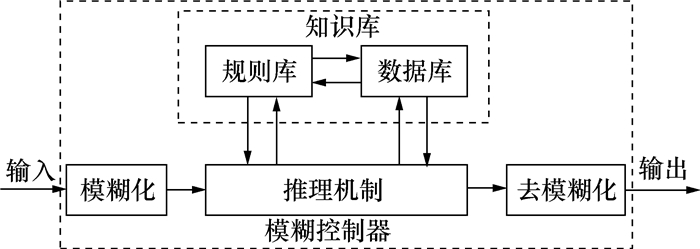图 3 模糊系统组成

 $\begin{array}{c}{R_{1} : \text { IF } x \text { is } A_{1} \text { and } y \text { is } B_{1}, \text { THEN } z \text { is } C_{1}}; \\ {\text { also } R_{2} : \text { IF } x \text { is } A_{2} \text { and } y \text { is } B_{2}, \text { THEN } z \text { is } C_{2}}; \\ {\vdots} \\ {\text { also } R_{n} : \text { IF } x \text { is } A_{n} \text { and } y \text { is } B_{n}, \text { THEN } z \text { is } C_{n}}.\end{array}$ (4)

3.2 模糊逻辑模型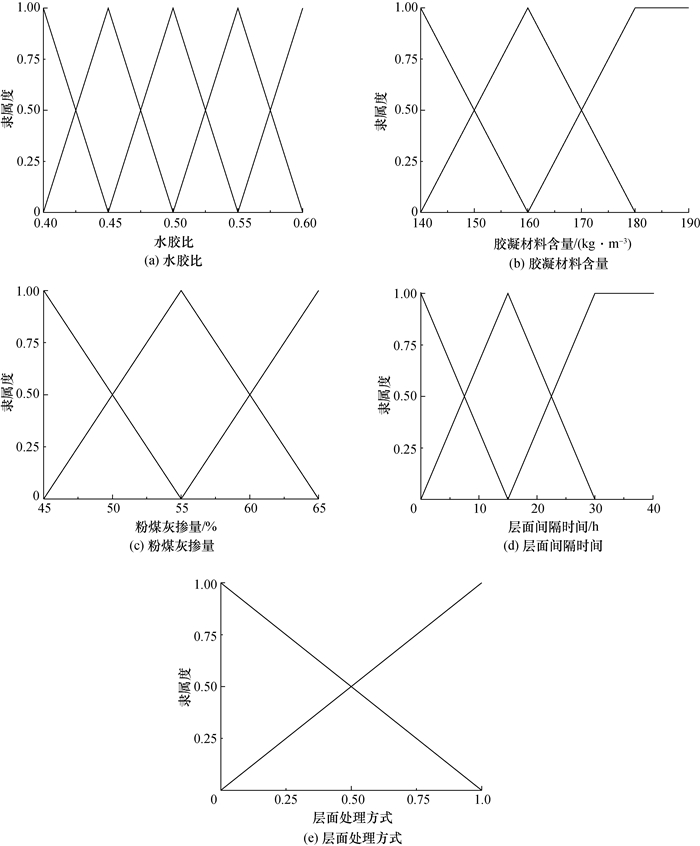图 4 输入变量对应的隶属度函数图 5 输出变量对应的隶属度函数

 $\begin{array}{c}{R_{i} \text { IF }\left(W \text { is } W_{i}\right) \text { and }\left(T \text { is } T_{i}\right) \text { and }\left(P \text { is } P_{i}\right)} \\ {\text { and }\left(H \text { is } H_{i}\right) \text { and }\left(M \text { is } M_{i}\right) \text { THEN }} \\ {\left(f^{\prime} \text { is } f_{i}^{\prime}\right) \text { and }\left(c^{\prime} \text { is } c_{i}^{\prime}\right)(i=1, 2, \cdots, 5)}.\end{array}$

4 结果与讨论

 $\operatorname{RMSE}=\sqrt{\frac{1}{m} \sum\limits_{i=1}^{m}\left(y_{i}^{\prime}-y_{i}\right)^{2}}.$ (5)

 $\mathrm{MAE}=\frac{1}{m} \sum\limits_{i=1}^{m}\left|y_{i}^{\prime}-y_{i}\right|.$ (6)

 $\mathrm{MAPE}=\frac{1}{m} \sum\limits_{i=1}^{m} \frac{\left|y_{i}^{\prime}-y_{i}\right|}{y_{i}}.$ (7)

 $\mathrm{MAX}_{-} \mathrm{RE}=\max \left(\frac{\left|y_{i}^{\prime}-y_{i}\right|}{y_{i}} \times 100 \%\right).$ (8)

 $R^{2}=1-\frac{\sum\limits_{i=1}^{m}\left(y_{i}^{\prime}-y_{i}\right)^{2}}{\sum\limits_{i=1}^{m}\left(\overline{y}_{i}-y_{i}\right)^{2}}.$ (9)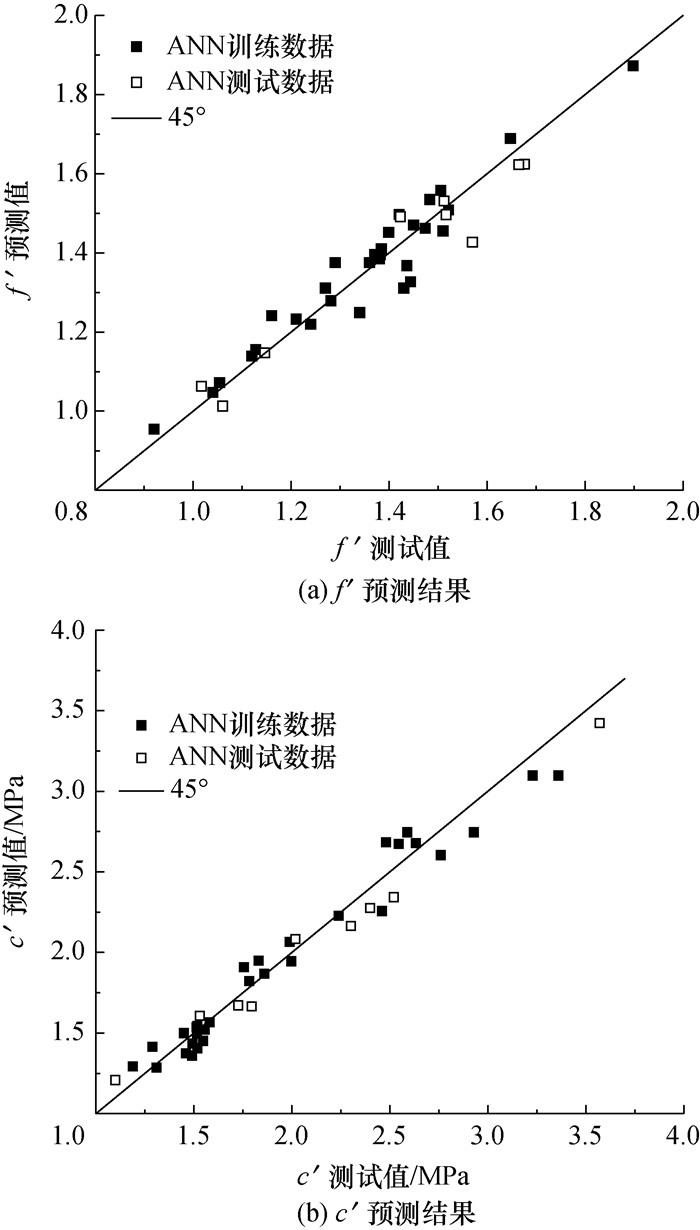图 6 室内试验人工神经网络预测结果图 7 室内试验模糊逻辑预测结果

 模型 数据 RMSE MAE MAPE MAX_RE/% R2 人工神经网络 训练 f′ 0.05 0.04 3.05 8.34 0.92 数据 c′ 0.12 0.09 4.72 9.71 0.96 测试 f′ 0.02 0.01 2.97 4.78 0.97 数据 c′ 0.06 0.02 5.63 9.82 0.95 模糊逻辑 训练 f′ 0.10 0.09 6.42 14.36 0.71 数据 c′ 0.19 0.15 7.41 20.60 0.92 测试 f′ 0.05 0.02 7.36 14.25 0.81 数据 c′ 0.06 0.03 6.63 20.00 0.86图 8 原位试验人工神经网络预测结果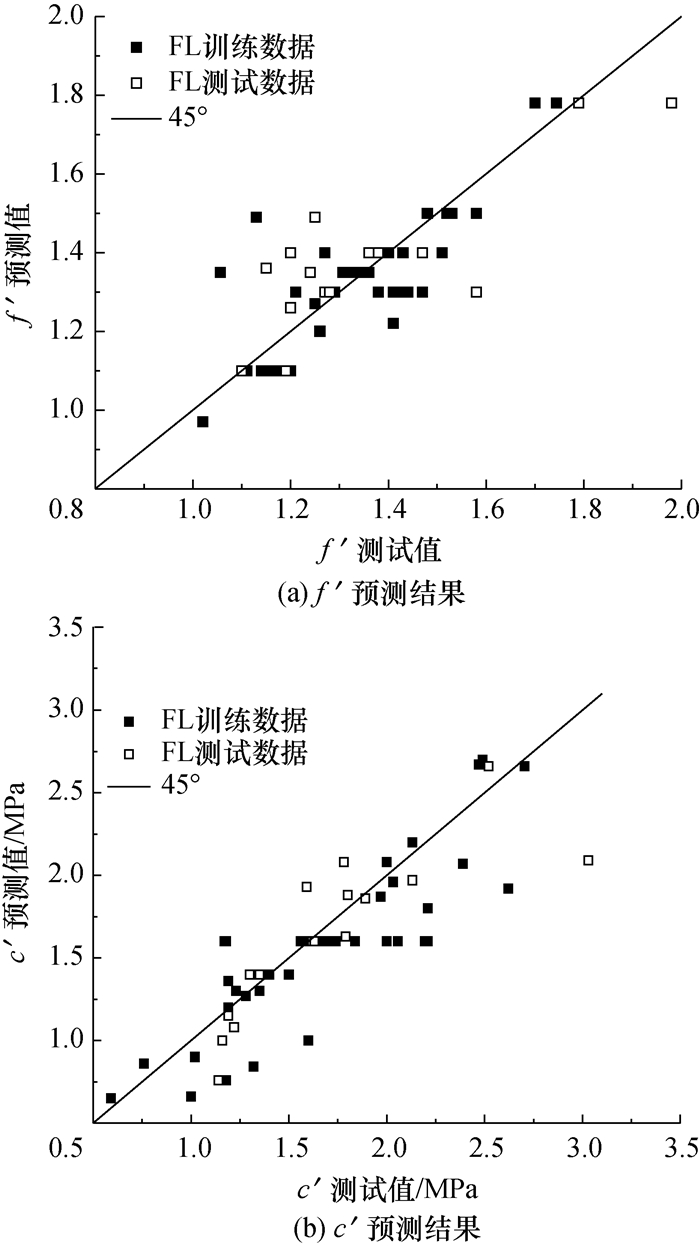图 9 原位试验模糊逻辑预测结果

 模型 数据 RMSE MAE MAPE MAX_RE/% R2 人工神经网络 训练 f′ 0.13 0.12 7.55 19.07 0.43 数据 c′ 0.31 0.26 13.34 35.59 0.72 测试 f′ 0.12 0.08 11.24 23.91 0.51 数据 c′ 0.20 0.11 11.53 21.73 0.70 模糊逻辑 训练 f′ 0.12 0.10 6.22 31.86 0.59 数据 c′ 0.34 0.28 14.09 37.50 0.66 测试 f′ 0.10 0.05 7.31 19.20 0.61 数据 c′ 0.21 0.10 10.87 33.51 0.68

5 结论

1) 碾压混凝土坝层面结合能力的影响因素众多，而且是综合作用的，使得层间结合能力的评估结果与影响因素之间表现出很强的非线性，不适合用传统的数学方法进行分析。

2) 人工神经网络是模拟人脑思维方式的一种数学模型，具有自学习功能。模糊逻辑使机器能模拟人脑的感知、推理等智力行为，在此基础上产生模糊控制。因此，神经网络和模糊逻辑系统为解决碾压混凝土坝层间抗剪断强度影响因素的非线性问题提供了有效的手段。

3) 本文通过分析常温条件下90 d龄期的碾压混凝土坝测试数据，考虑水胶比、胶凝材料含量、粉煤灰掺量、层面间隔时间和层面处理方式5个影响因素，分别对室内试验和原位抗剪试验数据进行了人工神经网络和模糊系统建模。

4) 模型结果显示：人工神经网络和模糊系统均能较准确地预测室内试验的强度参数，而对原位抗剪试验的预测结果较差，只能反映大致的规律。这是由于原位抗剪试验受气候条件的影响较大、不同工程的测试结果差异较大导致的。

5) 人工神经网络的预测结果较模糊系统更为准确，这可能是由于模糊系统的隶属度函数确定和模糊分割更依靠人的经验并具有较大的随机性。

6) 随着样本数量的不断积累，人工神经网络和模糊系统模型都可以动态地在新样本的基础上进行学习，通过调整已有的规则使模型的输出值更加接近新样本，从而提高模型的预测精度和应用范围，因此具有广泛的工程应用前景和实用价值。

  田育功. 碾压混凝土快速筑坝技术[M]. 北京: 中国水利水电出版社, 2010. TIAN Y G. Rapid dam construction technology for roller compacted concrete dams[M]. Beijing: China Water & Power Press, 2010. (in Chinese)  张严明, 王圣培, 潘罗生. 中国碾压混凝土坝20年[M]. 北京: 中国水利水电出版社, 2006. ZHANG Y M, WANG S P, PAN L S. 20 years of roller compacted dams in China[M]. Beijing: China Water & Power Press, 2006. (in Chinese)  张建文, 文家海, 申茂夏. 龙滩水电站碾压混凝土重力坝施工与管理[M]. 北京: 中国水利水电出版社, 2007. ZHANG J W, WEN J H, SHEN M X. Longtan Hydropower Station construction and management of RCC gravity dam[M]. Beijing: China Water & Power Press, 2007. (in Chinese)  石妍, 方坤河, 高钟伟. 碾压混凝土强度特性的研究[J]. 水力发电学报, 2006, 25(1): 85-89. SHI Y, FANG K H, GAO Z W. Research on strength of roller compacted concrete[J]. Journal of Hydroelectric Engineering, 2006, 25(1): 85-89. DOI:10.3969/j.issn.1003-1243.2006.01.018 (in Chinese)  王怀亮, 宋玉普. 多轴应力条件下碾压混凝土层面抗剪强度试验研究[J]. 水利学报, 2011, 42(9): 1095-1101, 1109. WANG H L, SONG Y P. Mechanical properties of roller compacted concrete under multiaxial stress state[J]. Journal of Hydraulic Engineering, 2011, 42(9): 1095-1101, 1109. (in Chinese)  林长农, 金双全, 涂传林. 龙滩有层面碾压混凝土的试验研究[J]. 水力发电学报, 2001(3): 117-129. LIN C N, JIN S Q, TU C L. Experiment researches on RCC with layer surfaces for Longtan project[J]. Journal of Hydroelectric Engineering, 2001(3): 117-129. DOI:10.3969/j.issn.1003-1243.2001.03.014 (in Chinese)  刘数华, 曾力, 吴定燕. 提高碾压混凝土层面粘结性能的试验研究[J]. 水力发电学报, 2004, 23(3): 61-65. LIU S H, ZENG L, WU D Y. Testing research on improvement of layer adhesion of roller compacted concrete[J]. Journal of Hydroelectric Engineering, 2004, 23(3): 61-65. DOI:10.3969/j.issn.1003-1243.2004.03.012 (in Chinese)  黄志强, 宋玉普, 王学志. 碾压混凝土层面拉伸破坏试验研究[J]. 大连理工大学学报, 2007, 47(3): 387-391. HUANG Z Q, SONG Y P, WANG X Z. Experimental research on tensile failure process in rolled compacted concrete layer[J]. Journal of Dalian University of Technology, 2007, 47(3): 387-391. DOI:10.3321/j.issn:1000-8608.2007.03.016 (in Chinese)  SARIDEMIR M, TOPÇU İ B, ÖZCAN F, et al. Prediction of long-term effects of GGBFS on compressive strength of concrete by artificial neural networks and fuzzy logic[J]. Construction and Building Materials, 2009, 23(3): 1279-1286. DOI:10.1016/j.conbuildmat.2008.07.021  PALA M, ÖZBAY E, ÖZTAŞ A, et al. Appraisal of long-term effects of fly ash and silica fume on compressive strength of concrete by neural networks[J]. Construction and Building Materials, 2007, 21(2): 384-394.  TOPÇU İ B, SARIDEMIR M. Prediction of compressive strength of concrete containing fly ash using artificial neural networks and fuzzy logic[J]. Computational Materials Science, 2008, 41(3): 305-311.  TOPÇU İ B, SARIDEMIR M. Prediction of mechanical properties of recycled aggregate concretes containing silica fume using artificial neural networks and fuzzy logic[J]. Computational Materials Science, 2008, 42(1): 74-82.  KEWALRAMANI M A, GUPTA R. Concrete compressive strength prediction using ultrasonic pulse velocity through artificial neural networks[J]. Automation in Construction, 2006, 15(3): 374-379. DOI:10.1016/j.autcon.2005.07.003  ÖZTAŞ A, PALA M, ÖZBAY E, et al. Predicting the compressive strength and slump of high strength concrete using neural network[J]. Construction and Building Materials, 2006, 20(9): 769-775. DOI:10.1016/j.conbuildmat.2005.01.054  TAYFUR G, ERDEM T K, KIRCA Ö. Strength prediction of high-strength concrete by fuzzy logic and artificial neural networks[J]. Journal of Materials in Civil Engineering, 2014, 26(11): 04014079. DOI:10.1061/(ASCE)MT.1943-5533.0000985  石妍, 方坤河.碾压混凝土耐久性的神经网络预测方法[C]//第五届碾压混凝土坝国际研讨会论文集.贵阳: 中国水利学会, 中国水力发电工程学会, 2007. SHI Y, FANG K H. Neural network prediction method for durability of roller compacted concrete[C]//Proceedings of the International Symposium on Roller Compacted Concrete Dams. Guiyang: Chinese Hydraulic Engineering Society, China Society for Hydropower Engineering, 2007. (in Chinese) http://www.wanfangdata.com.cn/details/detail.do?_type=conference&id=6564628  李国勇, 杨丽娟. 神经·模糊·预测控制及其MATLAB实现[M]. 第3版. 北京: 电子工业出版社, 2013. LI G Y, YANG L J. Neural network·fuzzy logic·predictive control and realization by MATLAB[M]. 3rd ed. Beijing: Publishing House of Electronics Industry, 2013. (in Chinese)  毛剑英, 何先莉. 人工神经网络在化学和环境科学中应用的若干问题[J]. 中国环境监测, 2001, 17(5): 48-50. MAO J Y, HE X L. The problems of application of artificial neural networks in chemistry and environmental science[J]. Environmental Monitoring in China, 2001, 17(5): 48-50. DOI:10.3969/j.issn.1002-6002.2001.05.017 (in Chinese)  王宏硕. 碾压混凝土坝层面抗剪断强度的探讨[J]. 湖北水利发电, 1995(3): 17-21, 31. WANG H S. Discussion on shear strength of roller compacted concrete dams[J]. Hubei Water Power, 1995(3): 17-21, 31. (in Chinese)  王迎春.碾压混凝土层面结合特性和质量标准研究[D].杭州: 浙江大学, 2007. WANG Y C. Study on specific characteristics and qualification requirements of lift joints in roller compacted concrete[D]. Hangzhou: Zhejiang University, 2007. (in Chinese) http://www.wanfangdata.com.cn/details/detail.do?_type=degree&id=Y1271175